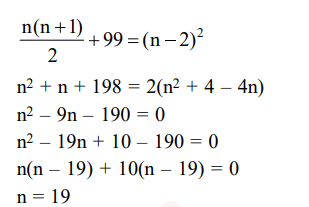# Some identical balls are arranged in rows to form an equilateral triangle.`
Question:

Some identical balls are arranged in rows to form an equilateral triangle. The first row consists of one ball, the second row consists of two balls and so on. If 99 more identical balls are addded to the total number of balls used in forming the equilaterial triangle, then all these balls can be arranged in a square whose each side contains exactly 2 balls less than the number of balls each side of the triangle contains. Then the number of balls used to form the equilateral triangle is :-

1. 190

2. 262

3. 225

4. 157

Correct Option: 1

Solution: